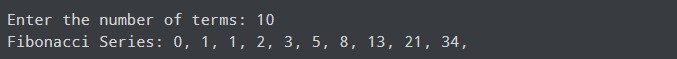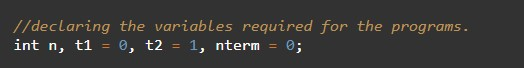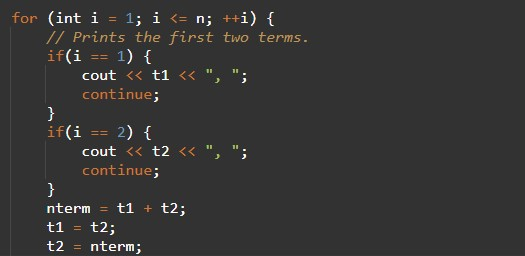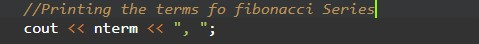# C++ Programs to Generate Fibonacci Series

In this tutorial you will learn about the C++ Programs to Generate Fibonacci Series and its application with practical example.

## C++ Programs to Generate Fibonacci Series

In this tutorial, we will learn to create a C++ program that will Reverse Array Element Using Function using C++ programming.

## Prerequisites

Before starting with this tutorial we assume that you are best aware of the following C++ programming topics:

• Operators in C++ Programming.
• Basic Input and Output function in C++ Programming.
• Basic C++ programming.
• For loop in C++ Programming.
• Functions in C++ Programming.

## What is Fibonacci Series?

The Fibonacci series is a series that is strongly related to Binet’s formula (golden ratio). in the Fibonacci series, the series starts from 0, 1 and then the third value is generated by adding those numbers,

## Programs to Generate Fibonacci Series:-

In this program, we take the number of elements for the series from the user. Then we will generate the Fibonacci series from the below program code.

## Program:-

Program to Generate Fibonacci Series

## Output:-In the above program, we have first initialized the required variable• t1 = it will hold the integer value.
• t2 = it will hold the integer value.
• nterm = it will hold the integer value.
• n = it will hold the integer value.
• i = it will hold the integer value to control the array.

Generating the fibonacci series.Program Code for Printing the series.In this tutorial we have learn about the C++ Programs to Generate Fibonacci Series and its application with practical example. I hope you will like this tutorial.